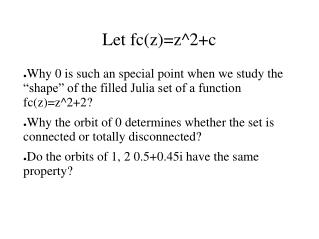DownloadDownload PresentationLet fc(z)=z^2+c

# Let fc(z)=z^2+c

Télécharger la présentation## Let fc(z)=z^2+c

- - - - - - - - - - - - - - - - - - - - - - - - - - - E N D - - - - - - - - - - - - - - - - - - - - - - - - - - -
##### Presentation Transcript

1. Let fc(z)=z^2+c • Why 0 is such an special point when we study the “shape” of the filled Julia set of a function fc(z)=z^2+2? • Why the orbit of 0 determines whether the set is connected or totally disconnected? • Do the orbits of 1, 2 0.5+0.45i have the same property?

2. For all c, 0 is a critical value of the funcion fc(z)=z^2+c. This property is what makes 0 “special”. The orbit of 0

3. The dynamics of all orbits is characterized by particular orbits – the orbits of the critical values. Typically, critical points are quadratic. Hence, when one considers a family of functions, the way the dynamics of this family should be modeled by the how the dynamics of family with one quadratic critical point varies. In general,

4. In this sense, the family fc(z)=z^2+c, where c varies over the complex plane, is a “model” for the dynamics of many “most” families of complex maps. Question:

5. This is way the Mandelbrot set appear in the family of functions we saw last class. There, 0 was also a critical value for all the maps in the family we studied.

6. In the Mandelbrot set, nature (or is it mathematics) provides us with a powerful visual counterpart of the musical idea of 'theme and variation': the shapes are repeated everywhere, yet each repetition is somewhat different. It would have been impossible to discover this property of iteration if we had been reduced to hand calculation, and I think that no one would have been sufficiently bright or ingenious to 'invent' this rich and complicated theme and variations. Mandelbrot's words

7. It leaves us no way to become bored, because new things appear all the time, and no way to become lost, because familiar things come back time and time again. Because this constant novelty, this set is not truly fractal by most definitions; we may call it a borderline fractal, a limit fractal that contains many fractals. Compared to actual fractals, its structures are more numerous, its harmonies are richer, and its unexpectedness is more unexpected More...